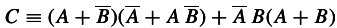Tags
#chapter-1 #has-images #jaynes_probability_theoryQuestion
This is just an example of a function. C = f(A, B)
How large is the class of new propositions thus generated?
two propositions, although they may appear entirely different when written out in the manner, are not different propositions from the standpoint of logic if they have the same truth value.
For A and B there 4 different inputs. TT, TF, etc. Size of the function: 2 -> 4, therefore there are 4^2 functions. A fixed number.
This easily generalizes to greater dimensions.

Tags
#chapter-1 #has-images #jaynes_probability_theoryQuestion
This is just an example of a function. C = f(A, B)
How large is the class of new propositions thus generated?
?

Tags
#chapter-1 #has-images #jaynes_probability_theory
Question
This is just an example of a function. C = f(A, B)
How large is the class of new propositions thus generated?
two propositions, although they may appear entirely different when written out in the manner, are not different propositions from the standpoint of logic if they have the same truth value.
For A and B there 4 different inputs. TT, TF, etc. Size of the function: 2 -> 4, therefore there are 4^2 functions. A fixed number.
This easily generalizes to greater dimensions.
If you want to change selection, open document below and click on "Move attachment"

#### pdfs

• owner: reshreshus - (no access) - JaynesProbabilityTheory.pdf, p13
• owner: hhhedw - (no access) - [概率论沉思录].Probability.Theory---The.Logic.Of.Science.pdf, p43

#### Summary

status measured difficulty not learned 37% [default] 0

No repetitions